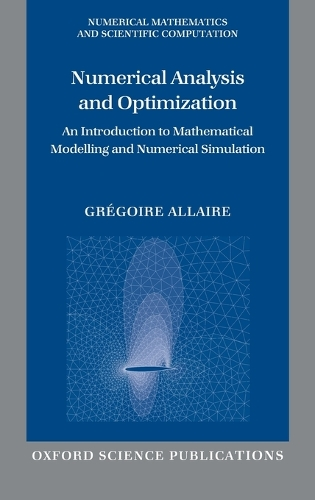•# Numerical Analysis and Optimization: An Introduction to Mathematical Modelling and Numerical Simulation - Numerical Mathematics and Scientific Computation (Hardback)

(author), (translator)
£135.00
Hardback 472 Pages / Published: 24/05/2007
• We can order this from the publisher

UK delivery within 3-4 weeks

This text, based on the author's teaching at Ecole Polytechnique, introduces the reader to the world of mathematical modelling and numerical simulation. Covering the finite difference method; variational formulation of elliptic problems; Sobolev spaces; elliptical problems; the finite element method; Eigenvalue problems; evolution problems; optimality conditions and algorithms and methods of operational research, and including a several exercises throughout, this is an ideal text for advanced undergraduate students and graduates in applied mathematics, engineering, computer science, and the physical sciences.

Publisher: Oxford University Press
ISBN: 9780199205219
Number of pages: 472
Weight: 824 g
Dimensions: 240 x 163 x 30 mm